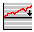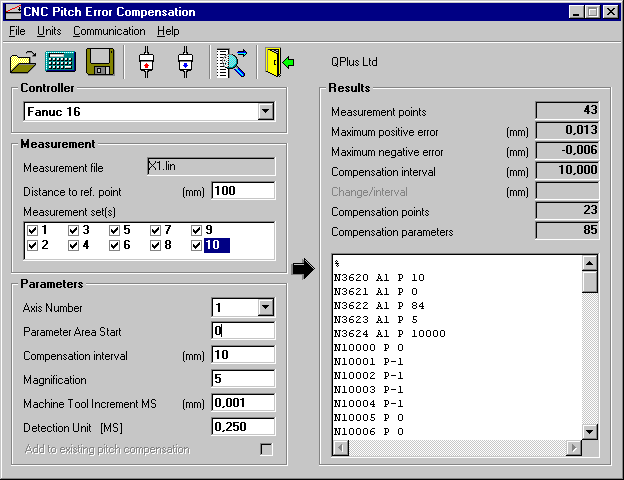## CNCcomp - Fanuc 16/18/21/160i/180i/210i CCNCcomp

#### Measurement File Options

Measurement file options are the same for all the controller types. Reference point is the distance between machine tool axes reference point and the start point of the measurement. If measurement file includes several measurement runs (sets) they all are listed in a Measurement set(s) listbox. It is possible to select just one or several sets for the compensation. If more than one set is selected the average of all selected measurement sets are used for the compensation.

#### Parameters Options

Axis Number
Axis number specifies which axes is to be compensated. The compensation file parameter options are changed accordingly.

Parameter Area Offset
Parameter address offset is added to the parameter address starting number. This is how different axes compensation parameter areas are separated. The maximum parameter address is 11023

Compensation Interval
Compensation interval defines how many compensation points are needed. By decreasing the interval smaller compensation steps are needed.

Magnification
Magnification value multiplies the used compensation step. If the magnification is 1 then the compensation step is the same as detection unit.

Machine Tool Increment
Machine tool increment is the used linear scale accuracy for the machine tool. Increment can be read from a downloaded parameter file (N1004).

Detection Unit
Detection unit is the unit by which compensation is made. So if the detection unit is 0.00025 mm and compensation is 2 and magnification is 5 then compensation at that point is 0,00025 * 2 * 5 = 0,0025

If existing pitch compensation file is read from NC, the new compensation can be actualised cumulative to old ones. If you want to measure and compensate without clearing the old compensations, read first the old values to PC, put a cross in this selection and measure machine tool compensations set active.

With this controller type, it is possible to read the current machine tool increment system from a file, if the current parameter area is downloaded from the controller. Parameter N1004 bit 7 and 8 defines the machine tool increment system in a following matter:

 Bit Machine tool Increment 00 IS-B 0,001 10 IS-C 0,0001 01 IS-A 0,01

Also the Detection unit is read from the file. Detection unit is read from a parameter N1820:

If N1820 is 2-96 then Detection unit = N1004/(N1820/2)
If N1820 is 102-127 then Detection unit = N1004/(1/(N1820-100))

#### Calculation

The calculation is done by pressing the CALCULATE toolbar button. Maximum value for compensation is 7 on each compensation point. If you get a message: "Compensation value over 7", you must either decrease compensation interval or increase magnification.

#### Results

Calculation results are shown on the right side of the form. The actual compensation file format is shown on the lower right corner of the window.

#### Saving the compensation parameter file

Compensation parameter file is saved by pressing the SAVE toolbar button. After this the save file dialog is opened.

#### Sample screen